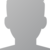Existing players used to logging in with their character name and moo password must signup for a website account.
 - robotdogfighter 42s - Baguette 8m waow - gssmr 1h a Mench 35m Doing a bit of everything. - BelladonnaRP 56s - JMo 6s Nothin' to see here. - Hek 26m youtu.be/iI1zl-7nVS4 - Soft_kitty 7m - Coris5271 1h - zeezenfrozen 36m mood: https://youtu.be/Hed0GAtoXk4 - Xanithar 5h - Slyter 1h And 23 more hiding and/or disguised
Connect to Sindome @ moo.sindome.org:5555 or just

# Part 4: Math 1 + 1 = ?

As you probablly noticed in the 99 Bottles of beer script, you can now 'decrement' (decrease by one) variables that are integers (numbers). You can also increment (increase by one) them with the 'increment' command.

You can also do math with the 'math' command.

The math command takes a result variable, a left hand operand, a operator, and a right hand operand, and sets the result to the result variable, with valid operators being + - * / (plus, minus, multiply, divide respectively).

It looks like this.

set "%a" 1
set "%b" 1
math "%c" = %a "+" %b
// %c is now 2
math "%d" = 2 "*" %c
// %d is now 4
math "%d" = %d "-" 1
// %d is now 3

You can check to see if two numbers are equal or not equal in the standard way:

if equals 1 %a
//do something
endif

if notequals %a %b
//do something
else
//do something else
endif

And finally you can get a random number between 1 and X by with 'random "%rand" X' where X is any positive number. If you want to make it negative afterwords, multiply it by -1.

Like this

random "%rand" 4
if equals 1 %rand
//do 1 here
elseif equals 2 %rand
//do 2 here
elseif equals 3 %rand
//do 3 here
elseif equals 4 %rand
//do 4 here
endif

If your confused as to why this is useful, take a look at the 99 Bottles of Beer script... You can do really dynamic things.